StatLect

Probit classification model (or probit regression)

This lecture deals with the probit model, a binary classification model in which the conditional probability of one of the two possible realizations of the output variable is equal to a linear combination of the inputs, transformed by the cumulative distribution function of the standard normal distribution.Model specification

Assume that a sample of data, for, is observed, where:

•is an output variable that can take only two values, eitheror(it is a Bernoulli random variable);

•is avector of inputs.

The conditional probability that the outputis equal to, given the inputs, is assumed to be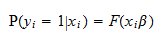whereis the cumulative distribution function of the standard normal distribution andis avector of coefficients.

Moreover, ifis not equal to, then it is equal to(no other values are possible), and the probabilities of the two values need to sum up to, so thatInterpretation

The interpretation of the probit model is very similar to that of the logit model. You are advised to read the comments about the interpretation of the latter in the lecture entitled Logistic classification model.

The probit model as a latent variable model

As in the case of the logit, also the probit model can be written as a latent variable model.

Define a latent variable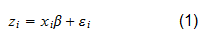whereis a random error term having a standard normal distribution. The outputis linked to the latent variable by the following relationship:We have that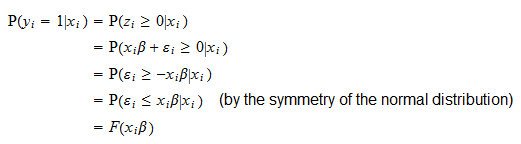so that the latent variable model specified by (1) and (2) assigns to the inputs the same conditional distributions assigned by the probit model.

Estimation by maximum likelihood

The vector of coefficientscan be estimated by maximum likelihood (ML).

We assume that the observationsin the sample are independently and identically distributed ( IID) and that hematrix of inputs defined byhas full rank.

In a separate lecture ( ML estimation of the probit model), we demonstrate that the ML estimatorcan be found (if it exists) with the following iterative procedure.

Starting from an initial guess of the solution(e.g.,), we generate a sequence of guesses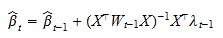is andiagonal matrix andis anvector. They are calculated as follows:

• compute• denote bythe probability density function of the standard normal distribution, and compute the entries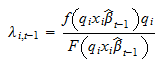of thevector• compute thediagonal matrixThe iterative procedure stops when numerical convergence is achieved, that is, when the difference between two successive guessesandis so small that we can ignore it.

Ifis the last step of the iterative procedure, then the maximum likelihood estimator isand its asymptotic covariance matrix iswhere.

As a consequence, the distribution ofcan be approximated by a normal distribution with mean equal to the true parameter and covariance matrix.

Hypothesis testing

When we estimate the coefficients of a probit classification model by maximum likelihood (see previous section), we can carry out hypothesis tests based on maximum likelihood procedures (e.g., Wald, Likelihood Ratio, Lagrange Multiplier) to test a null hypothesis about the coefficients.

Furthermore, we can set up a z test to test a restriction on a single coefficient:whereis the-th entry of the vector of coefficientsand.

The test statistic iswhereis the-th entry ofandis the-th entry on the diagonal of the matrix.

Sinceis asymptotically normal andis a consistent estimator of the asymptotic covariance matrix of,converges in distribution to a standard normal distribution (the proof is identical to the proof we have provided for the asymptotic normality of the z statistic in the lecture on the logit model).

By approximating the distribution ofwith its asymptotic one (a standard normal), we can derive critical values (depending on the desired size) and carry out the test.

The book

Most of the learning materials found on this website are now available in a traditional textbook format.

Glossary entries
Share